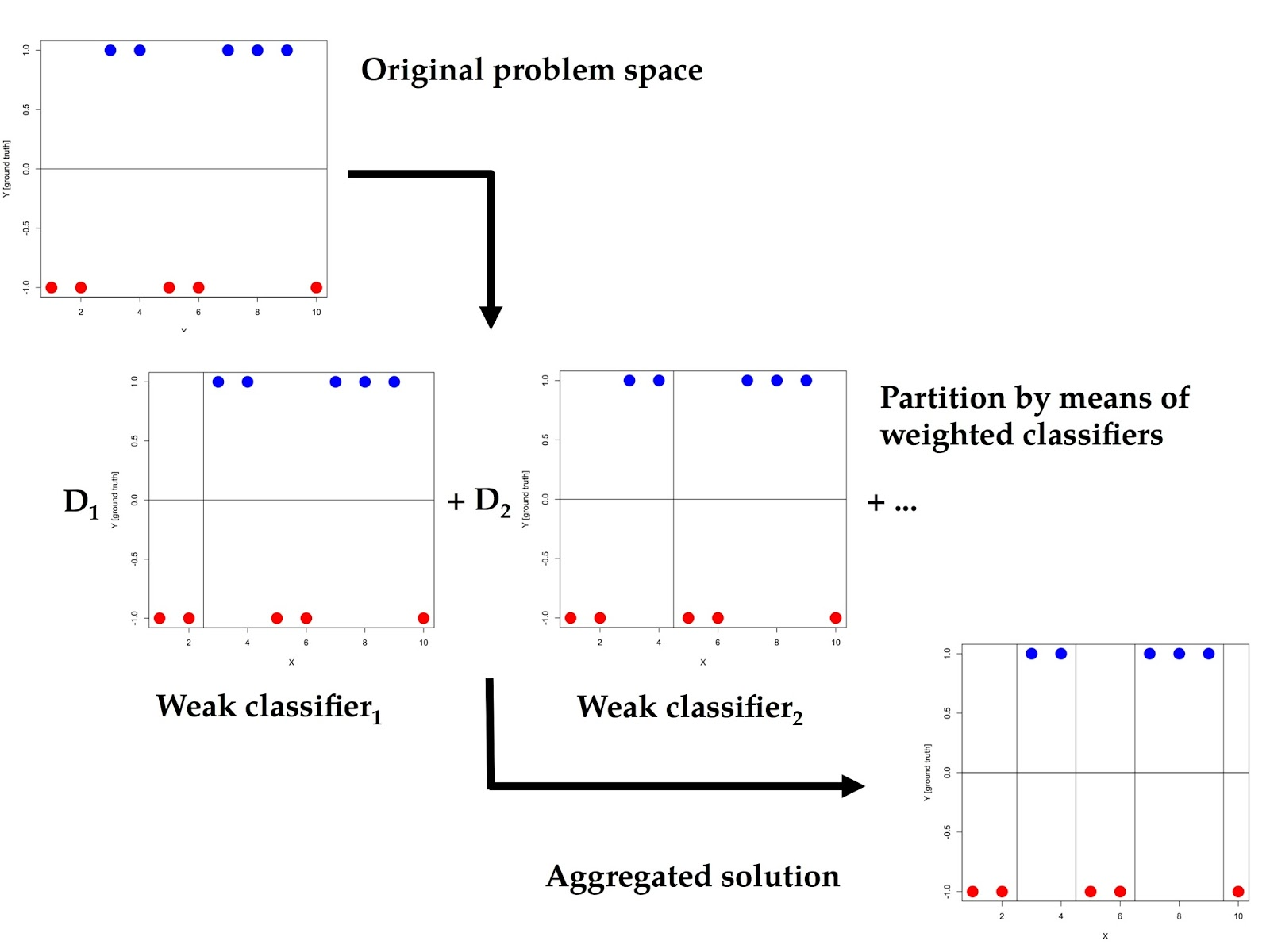### Boosting the accuracy rate by means of AdaBoost

Several machine learning techniques foster the cooperation between subsolutions for obtaining an accurate outcome by combining many of them. Michigan-style LCSs are one of these families. However, the most well known are those that implement Boosting, and AdaBoost is the most successful of them (or, at least, the most studied one and the first to implement the ideas of Boosting).

AdaBoost generates accurate predictions by combining several weak classifiers (also referred to as “the wisdom of the crowd”). The most outstanding fact about AdaBoost is that it is a deadly simple algorithm. The key of its success lies in the combination of many weak classifiers: these are very limited and their error rate is just slightly better than a random choice (hence their name). The typical implementation uses decision stumps (i.e., binary trees) that minimize the error between the prediction and the ground truth (that is, the desired outcome), so the classifiers have the form "if variable_1 < 3.145 then class = -1; otherwise class = +1". Another characteristic is that AdaBoost only handles two classes, namely {-1} and {+1}.

Its scheme is very simple: it trains classifiers that predict correctly a small part of the problem space and then it combines all these by using a weighting mechanism. Then, AdaBoost decides the class of the example by computing the sign (+1 or -1) of the aggregated predictions. Recall that the weights are computed based on the error achieved by the distinct weak predictors (see the image below).Figure 1. The learning process of AdaBoost. The Di's are the distinct weights applied to each weak classifier.

Boosting techniques have attracted my attention lately since these are the ones that provide the best results in the distinct Kaggle competitions. As usual, I implemented a little R code for playing a bit with this wonderful technique. It is listed in the following. Notice that I implemented the univariate version of the AdaBoost algorithm, keeping the code very simple and easy to understand and extend.